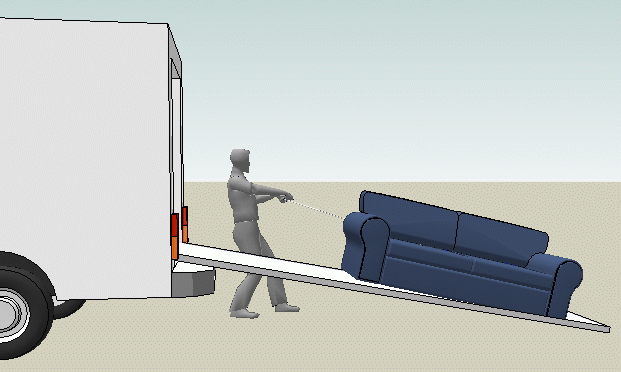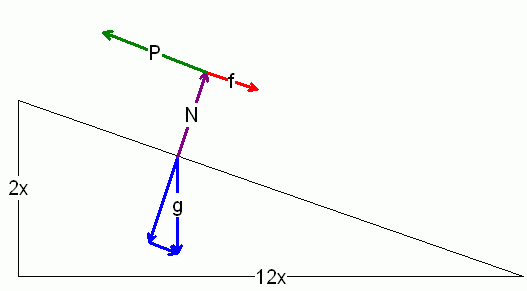SEARCH HOMEMath Central Quandaries & QueriesQuestion from Ron, a student: A body that weighs 540lbs is caused to slide up an inclined plane with a uniform velocity by a force that acts parallel to the plane. For each foot of horizontal distance, there is a vertical rise of 2in.  If the coefficient of sliding friction is 0.16, what force is required to move the body?Hi Ron.Let's draw the force diagram. Since the velocity is constant, net acceleration is zero and therefore net force is zero.The force g is the force due to gravity (use F = mg for this). N is the normal force, equal in magnitude to that portion of the gravitational force that is perpendicular to the incline plane. Both the friction force f and the pulling force P are parallel to the incline plane, as is the gravitational force component that is parallel to the incline plane.

I have labelled the sides 2x and 12x because the dimensions given indicate these proportions for the triangle.

Look at the diagram carefully and you will see similar triangles. Use a trig function to figure out the angles involved. Use these angles to calculate the perpendicular and parallel components of the gravitational force. Use the normal force to determine the frictional force according to the equation F = μN. Finally, use the fact that the net force is zero to calculate the pulling force needed to counteract the two forces (friction and gravitation) that are resisting it.

Hope this helps,
Stephen La Rocque.Math Central is supported by the University of Regina and The Pacific Institute for the Mathematical Sciences.